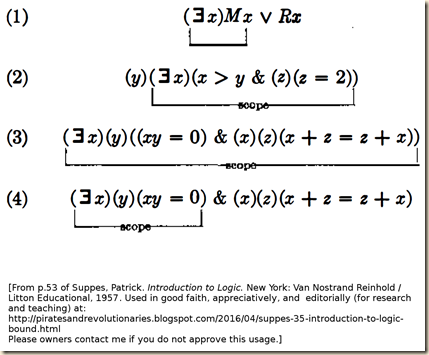## 4 Apr 2016

### Suppes (3.5) Introduction to Logic, "Bound and Free Variables", summary

[
Search Blog Here. Index-tags are found on the bottom of the left column.]

[Central Entry Directory]
[Logic & Semantics, Entry Directory]
[Patrick Suppes, entry directory]
[Suppes’ Introduction to Logic, entry directory]

[The following is summary. My commentary is in brackets.]

Summary of

Patrick Suppes

Introduction to Logic

Ch. 3 Symbolizing Everyday Language

§3.5 Bound and Free variables

Brief summary:
Atomic formulas are predicates followed or flanked by the appropriate number of terms as arguments. A formula more generally is either an atomic formula or a more complex formula built by adding or being combined by operators (–, &, ∨, →, ↔) or quantifiers ([the universal quantifier has no symbol before the variable here], ∃). The scope of the quantifier is the smallest formula following it, when that formula has no parentheses. Or it is whatever lies within the left-most parenthesis following the quantifier(s) and its right- sided partner. A variable in a formula is bound when it falls within the scope of the quantifier that uses it, and it is free otherwise. A sentence is a formula with no free variables in it.

Summary

When there is a variable in a formula, it is either controlled by a quantifier or it is not. We first note that an atomic formula “is a predicate followed or flanked by the appropriate number of terms as arguments” (52). The following expressions, then, are atomic formulas.

Ra
x + y = z
x
is blue

Suppes then gives the following recursive definition for formulas:

(a) Every atomic formula is a formula.
(b) If S is a formula, then (S) is a formula.
(c) If R and S are formulas then (R) & (S), (R) ∨ (S), (R) → (S), and (R) ↔ (S) are formulas.
(d) If R is a formula and v is any variable then (v)(R) and (∃v)(R) are formulas.
(e) No expression is a formula unless its being so follows from the above rules.

Thus x = y → ∨(x) ↔ is not a formula but rather a “meaningless string of symbols” (52).

Suppes now defines the scope of a quantifier:

DEFINITION. The SCOPE of a quantifier occurring in a formula is the quantifier together with the smallest formula immediately following the quantifier.
(53)

Then Suppes offers these examples.[I do not entirely understand the notion of “the smallest formula immediately following the quantifier.” It seems the idea is the following. The quantifier will have a formula or a group of formulas following it. The formula(s) will either have parentheses or it will not. If there are no parentheses, then the scope is merely the smallest formula following the quantifier, as in sentence 1. However, when there are parentheses, that formula’s left-most parentheses following the quantifier(s) and its right-sided partner determine the limits of the quantifier’s scope. So compare sentences 3 and 4 above. The reason why the (y)’s scope in 3 is the whole thing is because the left-most parenthesis coming right after it, and that parenthesis’s right-sided partner, enclose the entire formula. However in sentence 4, the left-most parenthesis following the quantifier (y) and its right-sided partner only enclose the left side of the conjunction.]

Suppes then defines bound occurrences of variables.

DEFINITION. An occurrence of a variable in a formula is BOUND if and only if this occurrence is within the scope of a quantifier using this variable.
(53)

In sentences 2-4 above, every occurrence of each variable is found within the scope of the quantifier using that variable, thus all instances of variables in those sentences are bound (53). [This seems to imply that in sentence 1, the x in Rx is not bound.]

Suppes then defines free variables.

DEFINITION. An occurrence of a variable is FREE if and only if this occurrence of the variable is not bound.
(53)

So we consider the formula:

(∃x)(x > y)

Here, “both occurrences of the variable ‘y’ are bound and the single occurrence of ‘y’ is free” (54). However, in sentence 1 above,

(∃x)(Mx Rx)

, “the first two occurrences of ‘x’ are bound and the third occurrence is free” (54). So

DEFINITION. A variable is a FREE VARIABLE in a formula if and only if at least one occurrence of the variable is free, and a variable is a BOUND VARIABLE in a formula if and only if at least one occurrence of the variable is bound.
(54)

As we can see from this definition, “a variable may be both free and bound in the same formula” (54). However, “any given occurrence of the variable is either bound or free but not both” (54). So we consider the formula

y > 0 ∨ (∃y)(y < 0)

Here, the variable y is found both free and bound. However, each instance of its appearing is only one or the other. In its first occurrence it is free [because no quantifier binds it]. However, its second and third occurrences are bound (54).

Now, a formula can be true or false only if it has no free variables. And a formula is a sentence so long as it is either true or false. Thus

DEFINITION. A SENTENCE is a formula which has no free variables.
(54)

This means that formulas 2-4 are sentences, but formula 1 is not (54).

Also, when we symbolize sentences from everyday language, we should make sure that the formulations contain no free variables (54).

Suppes, Patrick. Introduction to Logic. New York: Van Nostrand Reinhold / Litton Educational, 1957.

.